Russian Academy of Sciences Central Astronomical Observatory at Pulkovo SIXTH US/RUSSIAN SPACE SURVEILLANCE WORKSHOP August 22-26, 2005 Proceedings Edited by P. Kenneth Seidelmann and Victor K. Abalakin St. Petersburg, 2005<<< Contents

### A STUDY OF THE SURFACE ENVELOPING THE TRAJECTORY FAMILY OF PARTICLES ISOTROPICALLY EJECTED BY A SATELLITE EXPLOSION WITH ACCOUNT FOR THE ORBIT NODE AND PERICENTER MOTIONS

K. V. Kholshevnikov, S. A. Orlov

Astronomical Institute of St.Petersburg University, St.Petersburg, Petrodvoretz, Universitetsky ave. 28, 198504 RUSSIA
##### 1. INTRODUCTION

An explosion of an artificial satellite leads to the ejection of a mass of fragmentary particles into the cosmic space. Typical velocities of the ejecta are of the order of one km/s or less, so they remain on geocentric orbits T close to the supposedly circular orbit of the satellite. The emerged swarm fills out a domain D swept by family {T}. We are interested in studying the structure of the domain D, corresponding to the largest possible family {T}. It leads to an assumption of the ejecta in all directions. Now let’s imagine that we observe an isotropic ejection with all possible velocities smaller than the absolute value b.

Due to the inequality of orbital periods, the fragments will densely fill up the domain D'. To find its boundary S', it is sufficient to suppose the velocities to be equal to b. So, D' represents a dust complex appearing a few days after the explosion.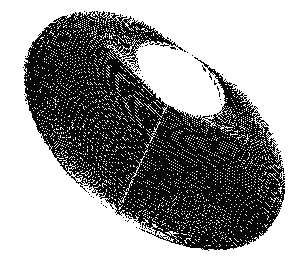Fig. 1. View of S', c = 0.3

We have analytically obtained the parametric equations of the boundary S', its properties having been examined in our previous paper . Topologically, S' is a torus with one conic point and one rectilinear constriction (see Fig. 1). In few months D' scatters due to the motion of the nodes and pericenters of particle orbits in the gravitation field of an oblate central planet, which yields the axisymmetric solid D with the boundary ^S. Topologically, D is a torus.

Here we have obtained the parametric equations of ^S and examined its properties for the case where the orbit of the exploded satellite lies in the equatorial plane of the central planet.
##### 2. PROPERTIES OF S

Similar to , we use the methods of the mathematical theory of catastrophes. The boundary ^S of D is the set of singular points of the mapping {T} → R3. Obviously, it is a body of revolution and topologically occurs to be a torus. Hence, it is sufficient to examine its section S by the plane xz.

The mathematical formalism, while obtaining the parametric equations of S, is rather cumbersome, so that we confine ourselves to giving the results.

The curve S is symmetric with respect to the x-axis. It consists of the convex oval S0 with two additional curvilinear triangles. These triangles are so small that they may be neglected (see Figs. 2 and 3).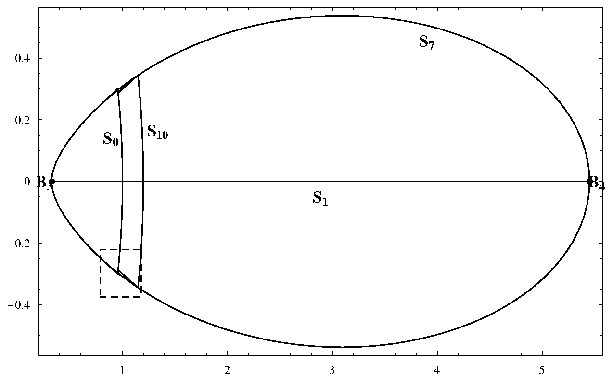Fig. 1. View of S, c = 0.3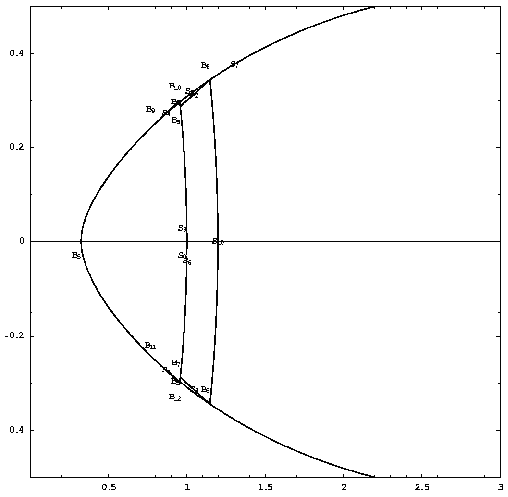Fig. 1. View of part of S, c = 0.3

The parametric equations of S0 are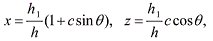with c = b expressed in units of the circular velocity of the satellite,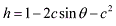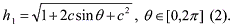The equations are not very complicated. We can easily examine their properties and find the following properties of S0.

The curve S0 is closed, bounded and symmetric with respect to the x-axis. The curve S0 is analytic (regular) without singular points. The curvature of S0 is negative, hence the curve S0 is convex.

The family {S0 (c)} represents the family of embedded ovals tending to a point as c → 0. If c → 0, then S to S0 and S0 tends to an ellipse with the 1:4 axis-ratio.
##### 3. CONCLUSIONS

We see that the boundary S of the section of D by the plane xz is an oval. If c is large, the oval is elongated backwards from the planet. If c is small, the oval is symmetrical representing the ellipse with the 1:4 axis-ratio.
##### REFERENCES

1. Kholshevnikov K.V., Orlov S.A. (2003) Enveloping Surface of Trajectory Family of Isotropically Ejected Particles Due to a Satellite Explosion. Proc. Fifth US/Russian Space Surveillance Workshop, Sept. 24-27, Pulkovo, St.Petersburg, Russia, pp. 183-191.

Размещен 30 ноября 2006.